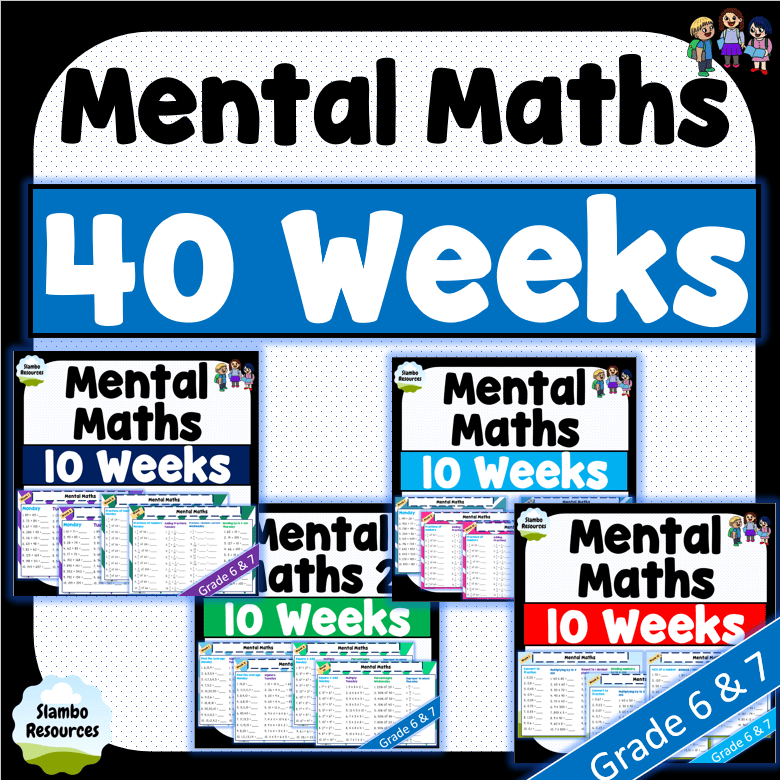## Mental Math Worksheets

Free mental math worksheets for students in grades 2 to 6. Mental maths is an important part of Mathematics and must be done daily. 10 minutes a day of mental maths a day and you will see significant improvement in your students’ ability to recall math facts. These worksheets can be used during daily math warm ups or as homework. They cover a variety of mathematical concepts including addition, subtraction, multiplication, division, fractions, decimals, ordering numbers, patterns, angles, shapes, percentages, algebra and much more! Click on the images below to download the mental math worksheets. For 5 tips on improving your students’ daily mental math skills, scroll to the bottom of the page. For more free mental math worksheets, check out Salamander Maths. They have a range of free mental math worksheets for all grades that are ready to print and be used in your classroom!### Bundle

A bundle of the 4 resources.

## 5 Ways to Improve Mental Maths

Mental maths is such an important part of Mathematics. It should be an integral part of your daily math lessons.
This text will outline 5 ways to improve your students’ mental math skills.

### 1. Daily Mental Maths

Mental maths is so important that is must be done daily. 10 minutes during your maths warm up or 10 minutes at home each night will have a significant affect on your child’s ability to recall math facts. Furthermore, daily repetition allows students to cement their understanding of new math concepts. Mental maths must be done daily!

### 2. Not Just Times Tables

Once you have taught a math concept, add it into your daily mental maths! Often, teachers and parents think mental maths is just about times tables. There are so many math concepts that can be added into mental math activities. You can add in fractions of numbers, identifying angles, adding fractions, ordering decimals, ordering fractions, multiplying & dividing by 10, 100 & 100, number patterns, algebra, adding & subtracting large numbers, shape properties and so much more! So, once you have taught your class a new concept, add it into your daily mental maths!